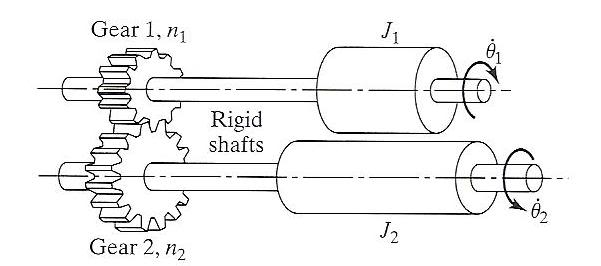# Finding equivalent mass moment of inertia for gear system

immel

## Homework Statement

Two masses, having mass moments of inertia J1 and J2, are placed on rotating rigid shafts that are connected by gears. If the numbers of teeth on gears 1 and 2 are n1 and n2, respectively, find the equivalent mass moment of inertia corresponding to theta_1.## Homework Equations

Kinetic energy of a rotating shaft = 1/2*J*theta_dot
J = 1/2*m*r^2
n1/n2 = theta_1/theta_2 ?????

## The Attempt at a Solution

This is for my mechanical vibrations class for ME majors. I assume I need to relate theta_dot_1 to theta_dot_2 somehow. I am also not sure what to do with the number of teeth. It seems as though I may be able to do something with the gear ratio, but I don't know where to begin relating things. Please help!

## Answers and Replies

Homework Helper
hi immel!(have a theta: θ and try using the X2 and X2 icons just above the Reply box)

don't you have cogs in your brain?the ratio of the number of turns equals the ratio of the number of teeth!immel
Thanks for the help tiny-tim.

So was I right with my assumption that n1/n2 = θ12 ?

I am confused about what variable I am solving for. In the problem statement "...find the equivalent mass moment of inertia corresponding to θ1." Does this mean I'm solving for θ1 or some product with the unit of mass*length2 (mass moment of inertia has these units)? J=1/2*m*r2 has units of mass*length2.

It's starting to look like I need to relate the kinetic energy equation and n1/n2 = θ12.

Homework Helper
hi immel!I am confused about what variable I am solving for. In the problem statement "...find the equivalent mass moment of inertia corresponding to θ1." Does this mean I'm solving for θ1 or some product with the unit of mass*length2 (mass moment of inertia has these units)? J=1/2*m*r2 has units of mass*length2.

It's starting to look like I need to relate the kinetic energy equation and n1/n2 = θ12.

i think by "equivalent" it means that if you pretend that you can only see one cog, and it's rotating at angular speed θ1', then you want a formula L = Jequivθ1', where L is the angular momentum for the whole system, and Jequiv can then be called the equivalent (or effective) mass moment of inertia for the whole systemimmel
I've come up with a solution, but using the equivalent kinetic energy method.

With the relation n1/n2 = θ12 = θ1'/θ2' (is this valid?), I solved for θ2' = (θ1'*n2)/n1

I have KE1=1/2*J11'2 and KE2=1/2*J2*[(θ1'*n2)/n1]2

I then have KEsystem = KE1 + KE2 where KEsystem = 1/2*Jeq1'2

Canceling out the 1/2 and θ1'2 I was left with Jeq = J1 + J2*(n2/n1)2

Sooo, how far off am I?

Homework Helper
I've come up with a solution, but using the equivalent kinetic energy method.

oh, that's probably right then!(i haven't come across equivalent moment of inertia before)
Canceling out the 1/2 and θ1'2 I was left with Jeq = J1 + J2*(n2/n1)2

Looks good!immel
Thanks tiny-tim! Much appreciated

jtfrizzy
I know this is about 2 years later but in case anyone comes across this looking for help I wanted to correct an error I noticed in the calculations. When making the relationship between number of teeth, [n][/1] and [n][/2] and angular velocity [θ'][/1] and [θ'][/2] they are inversely proportional. Namely, if [n][/1] < [n][/2] then [θ'][/1] > [θ'][/2]. If you think about it less teeth equals smaller diameter and when a constant torque is applied the smaller gear (and shaft coupled to it will spin faster than the gear with more teeth. Right? So the appropriate relations ship should between the two is:
[n][/1]/[n][/2]=[θ'][/2]/[θ'][/1]

NOTE: often both "n" and "N" are used in gear train calculations but refer to different things. "N" would be the RPMs of the gear and "n" equals the number of teeth of the gear. Adding diameter "d" onto the mix the following relationships all exist:

[d][/1]/[d][/2]=[n][/1]/[n][/2]=[N][/2]/[N][/1]=[θ'][/2]/[θ'][/1]

This is if, of course, the diameter and number of teeth are dirrectly dependent upon one another (which they are if your using gears in the real world)

jtfrizzy
Sorry about [x][/1] crap in the earlier post I guess I did the X_subscript wrong so I hope anyone reading it can get the drift